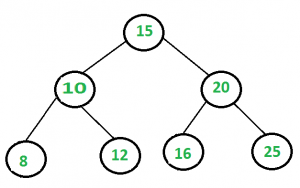# Sum of all nodes in a binary tree

Give an algorithm for finding the sum of all elements in a binary tree.In the above binary tree sum = 106.

## Recommended: Please solve it on “PRACTICE” first, before moving on to the solution.

The idea is to recursively, call left subtree sum, right subtree sum and add their values to current node’s data.

## C++

 `/* Program to print sum of all the elements of a binary tree */` `#include ` `using` `namespace` `std; ` ` `  `struct` `Node { ` `    ``int` `key; ` `    ``Node* left, *right; ` `}; ` ` `  `/* utility that allocates a new Node with the given key  */` `Node* newNode(``int` `key) ` `{ ` `    ``Node* node = ``new` `Node; ` `    ``node->key = key; ` `    ``node->left = node->right = NULL; ` `    ``return` `(node); ` `} ` ` `  `/* Function to find sum of all the elements*/` `int` `addBT(Node* root) ` `{ ` `    ``if` `(root == NULL) ` `        ``return` `0; ` `    ``return` `(root->key + addBT(root->left) + addBT(root->right)); ` `} ` ` `  `/* Driver program to test above functions*/` `int` `main() ` `{ ` `    ``Node* root = newNode(1); ` `    ``root->left = newNode(2); ` `    ``root->right = newNode(3); ` `    ``root->left->left = newNode(4); ` `    ``root->left->right = newNode(5); ` `    ``root->right->left = newNode(6); ` `    ``root->right->right = newNode(7); ` `    ``root->right->left->right = newNode(8); ` ` `  `    ``int` `sum = addBT(root); ` `    ``cout << ``"Sum of all the elements is: "` `<< sum << endl; ` ` `  `    ``return` `0; ` `} `

## Java

 `// Java Program to print sum of ` `// all the elements of a binary tree ` `class` `GFG ` `{ ` `static` `class` `Node  ` `{  ` `    ``int` `key;  ` `    ``Node left, right;  ` `} ` ` `  `/* utility that allocates a new  ` `   ``Node with the given key */` `static` `Node newNode(``int` `key)  ` `{  ` `    ``Node node = ``new` `Node();  ` `    ``node.key = key;  ` `    ``node.left = node.right = ``null``;  ` `    ``return` `(node);  ` `}  ` ` `  `/* Function to find sum  ` `   ``of all the elements*/` `static` `int` `addBT(Node root)  ` `{  ` `    ``if` `(root == ``null``)  ` `        ``return` `0``;  ` `    ``return` `(root.key + addBT(root.left) +  ` `                       ``addBT(root.right));  ` `}  ` ` `  `// Driver Code ` `public` `static` `void` `main(String args[]) ` `{  ` `    ``Node root = newNode(``1``);  ` `    ``root.left = newNode(``2``);  ` `    ``root.right = newNode(``3``);  ` `    ``root.left.left = newNode(``4``);  ` `    ``root.left.right = newNode(``5``);  ` `    ``root.right.left = newNode(``6``);  ` `    ``root.right.right = newNode(``7``);  ` `    ``root.right.left.right = newNode(``8``);  ` ` `  `    ``int` `sum = addBT(root);  ` `    ``System.out.println(``"Sum of all the elements is: "` `+ sum);  ` `}  ` `} ` ` `  `// This code is contributed by Arnab Kundu `

## Python3

 `# Python3 Program to print sum of all  ` `# the elements of a binary tree  ` ` `  `# Binary Tree Node  ` ` `  `""" utility that allocates a new Node  ` `with the given key """` `class` `newNode:  ` ` `  `    ``# Construct to create a new node  ` `    ``def` `__init__(``self``, key):  ` `        ``self``.key ``=` `key ` `        ``self``.left ``=` `None` `        ``self``.right ``=` `None` `         `  `# Function to find sum of all the element  ` `def` `addBT(root):  ` `    ``if` `(root ``=``=` `None``): ` `        ``return` `0` `    ``return` `(root.key ``+` `addBT(root.left) ``+`  `                       ``addBT(root.right))  ` ` `  `# Driver Code  ` `if` `__name__ ``=``=` `'__main__'``: ` `    ``root ``=` `newNode(``1``)  ` `    ``root.left ``=` `newNode(``2``)  ` `    ``root.right ``=` `newNode(``3``)  ` `    ``root.left.left ``=` `newNode(``4``)  ` `    ``root.left.right ``=` `newNode(``5``)  ` `    ``root.right.left ``=` `newNode(``6``)  ` `    ``root.right.right ``=` `newNode(``7``)  ` `    ``root.right.left.right ``=` `newNode(``8``)  ` ` `  `    ``sum` `=` `addBT(root)  ` ` `  `    ``print``(``"Sum of all the nodes is:"``, ``sum``) ` ` `  `# This code is contributed by ` `# Shubham Singh(SHUBHAMSINGH10) `

## C#

 `using` `System; ` ` `  `// C# Program to print sum of  ` `// all the elements of a binary tree  ` `public` `class` `GFG ` `{ ` `public` `class` `Node ` `{ ` `    ``public` `int` `key; ` `    ``public` `Node left, right; ` `} ` ` `  `/* utility that allocates a new   ` `   ``Node with the given key */` `public` `static` `Node newNode(``int` `key) ` `{ ` `    ``Node node = ``new` `Node(); ` `    ``node.key = key; ` `    ``node.left = node.right = ``null``; ` `    ``return` `(node); ` `} ` ` `  `/* Function to find sum   ` `   ``of all the elements*/` `public` `static` `int` `addBT(Node root) ` `{ ` `    ``if` `(root == ``null``) ` `    ``{ ` `        ``return` `0; ` `    ``} ` `    ``return` `(root.key + addBT(root.left) + addBT(root.right)); ` `} ` ` `  `// Driver Code  ` `public` `static` `void` `Main(``string``[] args) ` `{ ` `    ``Node root = newNode(1); ` `    ``root.left = newNode(2); ` `    ``root.right = newNode(3); ` `    ``root.left.left = newNode(4); ` `    ``root.left.right = newNode(5); ` `    ``root.right.left = newNode(6); ` `    ``root.right.right = newNode(7); ` `    ``root.right.left.right = newNode(8); ` ` `  `    ``int` `sum = addBT(root); ` `    ``Console.WriteLine(``"Sum of all the elements is: "` `+ sum); ` `} ` `} ` ` `  `// This code is contributed by Shrikant13 `

Output:

```Sum of all the elements is: 36
```

This article is contributed by Prakriti Gupta. If you like GeeksforGeeks and would like to contribute, you can also write an article using contribute.geeksforgeeks.org or mail your article to contribute@geeksforgeeks.org. See your article appearing on the GeeksforGeeks main page and help other Geeks.

Attention reader! Don’t stop learning now. Get hold of all the important DSA concepts with the DSA Self Paced Course at a student-friendly price and become industry ready.

My Personal Notes arrow_drop_up

Article Tags :
Practice Tags :

18

Please write to us at contribute@geeksforgeeks.org to report any issue with the above content.